Tamilnadu State Board New Syllabus Samacheer Kalvi 12th Accountancy Guide Pdf Chapter 4 Goodwill in Partnership Accounts Text Book Back Questions and Answers, Notes.

## Tamilnadu Samacheer Kalvi 12th Accountancy Solutions Chapter 4 Goodwill in Partnership Accounts

### 12th Accountancy Guide Goodwill in Partnership Accounts Text Book Back Questions and Answers

I Multiple Choice Questions

Question 1.
Which of the following statement is true?
(a) Goodwill is an intangible asset
(b) Goodwill is a current asset
(c) Goodwill is a fictitious asset
(d) Goodwill cannot be acquired
(a) Goodwill is an intangible assetQuestion 2.
Super profit is the difference between
(a) Capital employed and average profit
(b) Assets and liabilities
(c) Average profit and normal profit
(d) Current year’s profit and average profit
(c) Average profit and normal profit

Question 3.
The average rate of return of similar concerns is considered as
(a) Average profit
(b) Normal rate of return
(c) Expected rate of return
(d) None of these
(b) Normal rate of returnQuestion 4.
Which of the following is true?
(a) Super profit = Total profit / number of years
(b) Super profit = Weighted profit / number of years .
(c) Super profit = Average profit – Normal profit
(d) Super profit = Average profit × Years of purchase
(c) Super profit = Average profit – Normal profit

Question 5.
Identify the incorrecte pair
(a) Good will under average profit method – Average profit × Number of years of purchase
(b) Goodwill under Super profit method – Super profit × Number of years of purchase
(c) Goodwill under Annuity method – Average Profit × Present value annuity factor
(d) Goodwill under Weighted average profit method – Weighted average profit x Number of years of purchase
(c) Goodwill under Annuity method – Average Profit x Present value annuity factor

Question 6.
When the average profit is ₹ 25,000 and the normal profit ₹ 15,000 super profit is
(a) ₹ 25,000
(b) ₹ 5,000
(c) ₹ 10,000
(d) ₹ 15,000

Hints: Find out Super Profit
Super Profit = Average Profit – Normal Profit
= 25,000- 15,000
= ₹ 10,000
(c) ₹ 10,000Question 7.
Book profit of 2017 is ₹ 35,000; non -recurring income included in the profit is ₹ 1,000 and abnormal loss charged in the year 2017 was ₹ 2,000, then the adjusted profit is
(a) ₹ 36,000
(b) ₹ 35,000
(c) ₹ 38,000
(d) ₹ 34,000
Hints:

 Particulars 2017 ₹ Profit 35,000 Less: Non- recurring income 1,000 34,000 Add: Abnormal Loss in the year 2017 2,000 Profit after Adjustment 36,000

(a) ₹ 36,000

Question 8.
The total capitalised value of a business is ₹ 1,00,000; asset are ₹ 1,50,000 and liabilities ₹ 80,000. the value of goodwill as per the capitalisation method will be
(a) ₹ 40,000
(b) ₹ 70,000
(c) ₹ 1,00,000
(d) ₹ 30,000
Hints:
Goodwill = Total capitalized value of the business – Actual capital employed
i) Capital employed = Fixed Assets + Current assets – Current Liabilities
= 1,50,000-80,000
= ₹ 70,000
ii) Capitalized Value = ₹ 1,00,000
= 1,00,000 – 70,000
= ₹ 30,000
(d) ₹ 30,000Question 1.
What is goodwill?
Goodwill is the good name or reputation of the business which brings benefit to the business. It enables the business to earn more profit. It is the present value of a firm’s future excess earnings.

Question 2.
What has acquired goodwill?
Goodwill acquired by making payment in cash or kind is called acquired or purchased goodwill. When a firm purchases an existing business, the price paid of the purchase of such a business may exceed the net assets (Assets – Liabilities) of the business acquired. The excess of purchase consideration over the value of net assets acquired is treated as acquired goodwill.Question 3.
What is super profit?
Super profit is the excess of average profit over the normal profit of a business.
Super Profit = Average Profit – Normal Profit

Question 4.
What is normal rate of return?
:It is the rate at which profit is earned by similar business entities in the industry under normal circumstances.

Question 5.
State any two circumstances under which goodwill of a partnership firm is valued

1. When there is a change in the profit-sharing ratio
2. When a new partner is admitted into a firm
3. When an existing partner retires from the firm or when a partner dies.
4. When a partnership firm is dissolved.Question 1.
State any six-factor determining goodwill.

1. Profitability of the firm.
2. Favourable location of the business enterprise.
3. Good quality of goods or services offered.
4. Tenure of the business enterprise.
5. Efficiency of management.
6. Degree of competition.
7. Other factors.Question 2.
How is goodwill calculated under the super profits method?
Under these methods, super profit is the base for calculation of the value of goodwill. Super profit is the excess of average profit over the normal profit of a business.
Super profit = Average profit – Normal profit

Average profit is calculated by dividing the total of adjusted actual profits of certain number of year by the total number of such years. Normal profit is the profit earned by the similar business firms under normal conditions.
Normal profit = Capital employed × Normal rate of return
Capital employed = Fixed assets + Currents assets – Current liabilities.

Question 3.
How is the value of goodwill calculated under the capitalisation method?
Under these methods, super profit is the base for calculation of the value of goodwill. Super profit is the excess of average profit over the normal profit of a business.
Goodwill = Total capitalised value of the business – Actual capital employed
The total capitalised value of the business is calculation by capitalising the average profit on the basis of the normal rate of return.
Capitalised value of the business = $$\frac{\text { Average profit }}{\text { Normal rate of return }} \times 100$$
Actual capital employed = Fixed assets (excluding goodwill) + Current assets – Current liabilitiesQuestion 4.
Computer average profit from the following information.
2016: ₹ 8,000; 2017: ₹ 10,000; 2018: ₹ 9,000
Solution: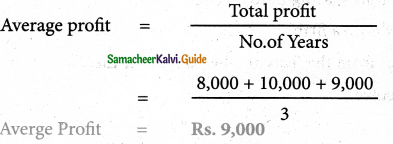Question 5.
Calculate the value of goodwill at 2 Years purchase of average profit when average profit is? 15,000.
Solution:
Goodwill = Average profit x No. of years of purchase
Goodwill = 15,000 x 2 Years
= Rs. 30,000

IV Exercises

Simple average profit method

Question 1.
The following are the profits of a firm in the last five years:
2014 : ₹ 10,000; 2015 : ₹ 11,000;
2016 :  ₹ 12,000; 2017 : ₹ 13,000; and 2018 : ₹ 14,000
Calculate the value of goodwill at 2 years purchase of average profit of five years.
Solution:
G/W = Average profit x No.of years of purchaseAverage profit: ₹ 12,000, Goodwill: ₹ 24,000Question 2.
From, following information, calculate the value of goodwillOn the basis of 3 years purchase of average profits of last four years.

 Year Result Amount 2015 Profit 5,000 2016 Profit 8,000 2017 Loss 3,000 2018 Profit 6,000

Solution:
G/W = Average profit x No.of years of purchaseGs/W = 4,000 × 3 years = Rs. 12,000
Average profit: ₹ 4,000; Goodwill: ₹ 12,000

Question 3.
From the following information relating to a partnership firm, find out the value of its goodwill based on 3 years purchas of average profits of the last 4 years.
(a) Profit of the years 2015, 2016, 2017 and 2018 are ₹ 10,000, ₹ 12,500, ₹ 12,000 and ₹ 11,500 respectively.
(b) The business was looked after by a partner and his fair remuneration amounts to ₹ 1,500, per year. This amount was not considered in the calculation of the above profits.
Solution:
G/W = Average profit = Average profit x No.of years of purchase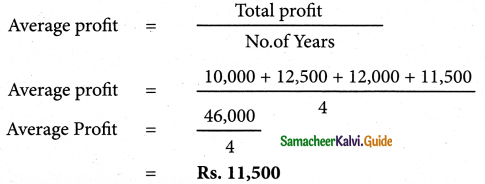₹ Average profit before adjusting four remuneration to the partner 11,500 Less : Remuneration to the partner 1,500 Average Profit 10,000

G/W Average profit × No.of years of purchase
= 10,000 × 3yrs
G/W = Rs. 30,000
Average profit: ₹ 10,000; Goodwill: ₹ 30,000Question 4.
From the following information relating to Sridevi enterprises, cal te the value of goodwill on the basis of 4 years pruchase of the average profits of 3 years.
(a) Profits for the years ending 31st December 2016, 2017 and 2018 were ₹ 1,75,000, ₹ 1,50,0 and ₹ 2,00,000 respectively.
(b) A non-recurring-income of ₹ 45,000 is include in the profits of the year 2016.
(c) The closing stock of the year 2017 was overvalued by ₹ 30,000.
Solution:Note : Overvaluationof cl. Stock in 2017 will results in over valuation of opening stock in 2018
Average Profit.
Average Profit. = $$\frac{1,30,000+1,20,000+2,30,00}{3}$$
Average Profit = $$\frac{4,80,000}{3}$$
= Rs. 1,60,000
= Average profit x No.of years of purchase
= 1,60,000 x 4 yrs
= Rs. 6,40,000
Average profit: ₹ 1,60,000; Goodwill: ₹ 6,40,000Question 5.
The following particulars are available in respect of the business carried on by a partnership firm:
(i) Profit earned : 2016 ₹ 25,000; 2017: ₹ 23,000 and 2018: ₹26,000.
(ii) Profit of 2016 includes a non-recurring income of ₹ 2,500.
(iii) Profit of 2017 is reduced by ₹ 3,500 due to stock destroyed by fire.
(iv) The stock was not insured. But, it is decided to insure the stock in future. The insurance premium is estimated to be ₹ 250 per annum.
you are required to calculate the value of goodwill of the firm on the basis of 2 years purchase of average profits of the last three years.
Solution: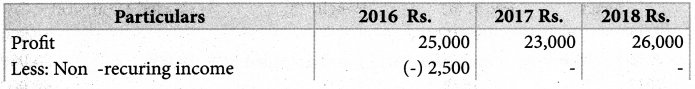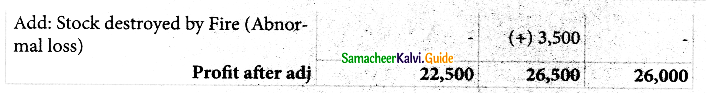Rs Average profit before adj insurance premium payable future 25000 Less : Ins, premium payable in future 250 Average Profit 24,750

G/W = Average profit × No.of years of purchase
G/W = 24,750 × 2 yrs
= Rs. 49,500
Average profit: ₹ 24,750; Goodwill: ₹ 49,500

Weighted average profit method.

Question 6.
Find out the value of goodwill at three years pruchase of weighted average profit of last four years.

 Year Profit Weight 2015 10,000 1 2016 12,000 2 2017 16,000 3 2018 18,000 4

Solution:
Calculation of Weighted Average profitWeighted profts = $$\frac{\text { Weighted profts }}{\text { Total of weights }}$$
Av.pft = $$\frac{1,54,000}{10}$$ = Rs. 15,400
G/w = Weighted Average profit × No. of yrs of purchase
G/w = 15,400 × 3yrs
= Rs. 46,200
Average profit: ₹15,400; Goodwill: ₹ 46,200Purchase of super profit method

Question 7.
From the following details, calculate the value o oodwill at 2 years purchase of super profit:
(a) Total assets of a firm are ₹ 5,00,000;
(b) The liabilities of the firm are ₹ 2,00,000
(c) Normal rate of return in this class of business is 12.5%.
(d) Average profit of the firm is ₹ 60,000.
Solution:
G/W = Super Profit x No.of years of purchase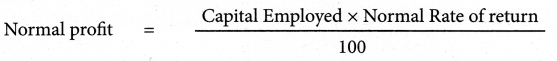Cap. Emp = Total Assets – Total liabilities
= 5,00,000 – 2,00,000
= Rs. 3,00,000
Normal Profit = $$\frac{3,00,000 \times 12.5}{100}$$
Rs. 37,500
Average Profit = Rs. 60,00
Super profit = Average Profit – Normal profit
= 60,000 – 37,500
= Rs. 22,500
G/w = 22,500 x 2 yrs
= Rs. 45,000
Super profit: ₹ 22,500; Goodwill: ₹ 45,000Question 8.
A partnership firm earned net profits during the last three years as follows:
2016 ₹ 20,000; 2017: ₹ 17,000 and 2018: ₹ 23,000.
The capital investement of the firm throghout the above mentioned period has been ₹ 80,000. Having regard to the risk involved, 15% is considered to the a fair return on capital employed in the business. Calculate the value of goodwill on the basis of 2 years purchase of super profit.
Solution:
G/W = Super Profit x No.of years of purchase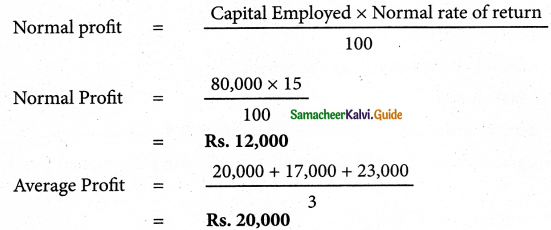Super profit = Average Profit – Normal profit
= 20,000 – 12,000
= Rs. 8,000
G/w = 8,000 x 2 yrs
= Rs. 16, 000
Super profit: ₹ 8,000; Goodwill: ₹ 16,000

Annuity Method:

Question 9.
From the following information, calculate the value of goodwill under annuity method:

 (i) Average profit ₹ 14,000 (ii) Normal profit ₹ 4,000 (iii) Normal rate of return 15% (iv) Years of purchase of goodwill 5

Present value of ₹ 1 for 5 years at 15% per annum as per the annuity table is 3.352
Solution:
G/W = Super Profit x Value of A nnuity
SuPer profit = Average Profit – Normal Profit
SuPer profit = 14,000- 4,000 = Rs. 10,000
G/w = 10.0 x 3.352 = Rs. 33,520
Super profit: ₹ 10,000; Goodwill: ₹ 33,520Capitalisation of super profit method:

Question 10.
Find out the value of goodwill by caitalising super profits:
(a) Normal Rate of Return 10%
(b) Profits for the last four years are ₹ 30,000, ₹ 40,000, ₹ 50,000, ₹ 45,000,
(c) A non-recurring income of ₹ 3,000 is included in the above mentioned profit of ₹ 30,000,
(d) Average capital employed is ₹ 3,00,000.
Solution:

 Rs. Profit for 1st year ₹ 30,000 Less: Non rec. Income 3000 Profit after adj 27,000= Rs. 30,000
Super profit – Average Profit – Normal Profit
Super Profit – 40,500 – 30,000
= Rs. 10,500Super Profit: ₹ 10,500; Good will: ₹ 1,05,000

Question 11.
From the following information, find out the value of goodwill by capitalisatio method:
(i) Average profit ₹ 20,000
(ii) Normal rate of return 10%
(iii) Tangible assets of the firm ₹ 2,20,000
(iv) Liabilities of the firm ₹ 70,000.
Solution:
G/W = Total capitalaised Value of the business – Actual cap.Employee Average ProfitActual cap employed = Tangible Assets – Liabilities of the firm.
= 2,20,000 – 70,000
= Rs. 1,50,000
G/w = 2,00,000 – 1,50,000
= Rs. 50,000
Total Capitalised value: ₹ 2,00,000; Net tangible assets: ₹ 1,50,000; Goodwill: ₹ 50,000### 12th Accountancy Guide Goodwill in Partnership Accounts Additional Important Questions and Answers

Question 1.
The excess of average profit over normal profit is
(a) Super profit
(b) Goodwill
(c) Undistributed profit
(a) Super profit

Question 2.
The goodwill that can be recoreded in the books of accounts and is shown on the assets side of the B/S is
(a) Acquired goodwill
(b) Self-Generated goodwil
(c) None of these two
(a) Acquired goodwill

Question 3.
How many methods are there for valuing G/W?
(a) 3
(b) 2
(c) 5
(a) 3Question 4.
Describe the nature of G/W.
The nature of goodwill can be described as follows:

• Goodwill is an intangible fixed asset. It is intangible because it has no physical existence. It cannot be seen or touched.
• It has a definite value depending on the profitability of the business enterprise.
• It cannot be separated from the business.
• It help in earning more profit and attracts more customers.
• It can be purchased or sold only when the business is purchased or sold in full or in part.Question 5.
Explain any 5 factors that determine the value of G/W
Factors determining the value of goodwill of a partnership firm
(i) Profitability of the firm
The profit earning capacity of the firm determines the value of its goodwill. A firm earning higher profits and having potential to generate higher profits in future will have higher value of goodwill.

(ii) Favourable location of the business enterprise
If the firm is located in a prominent place which is easily accessible to the customers, it can attract more customers. Its sales and profit will be higher when compared to a firm which is not located in a prominent place. Hence, it will ahve high value of goodwill.

(iii) Good equality of goods or services offered
If a firm is located in a prominent place which is easily accessible to the customers, it can attract more customers. Its sales and profit will be higher when compared to a firm which is not located in a prominent place. Hence, it will have high value of goodwill.

(iv) Tenure of the business enterprise
A firm which has caried on business for several years will have higher reputation among its customers as it is better known to the customers. Such a firm will have higher earnings and higher value of goodwill when compared to a new firm.

(v) Efficiency of management
A firm having efficient management will earn more profitsa and the value of its goodwill will be higher compared to a firm with less efficient managerial personnel.
(iii) It cannot be separated from the business.

Question 6.
What do you mean by self generated G/W?
It is the goodwill which is self generated by a firm based on features of the business such as favourable location, loya customers, etc. Such self-generated goodwill cannot be recorded in the books of accounts.Question 7.
How do you calculated Adjusted Profit?
+ Past expanse not required in the future.
– Past revenues not likely to be earned in the future
+ Additional income expected in the future
– Additional expenses expected to be paid in the future

Question 8.
How do you calculate G/W under weighted average profit method?
Weighted average profit method
Under this method, goodwill is calculated by mulitplying the weighted average profit by a certain number of years of purchase.
Goodwill = Weighted average profit × Number of years of purchase

In this method, weights are assigned to each year’s profit. Weighted profit is ascertained by multiplying the weights assigned with the respective years profit. The sum of the wieghted profits is divided by the sum of weights assigned to determine the weighted averagge profit.

Weighted average profit = $$\frac{\text { Total of weighted profits }}{\text { Total of weights }}$$Question 9.
What do you mean by Annuity Method of valuing G/w?
Under this method, value of goodwill is calculated by multiplying the super profit with the present value of annuity.
Goodwill = Super profit x Present value annuity factor
Present value annuity factor is the present value of annuity of rupee one at a given time. It can be found out from annuity table or by using formula.
Annuity factor = $$\frac{\mathrm{i}(1+\mathrm{i})^{\mathrm{n}}}{\mathrm{i}(1+\mathrm{i})^{\mathrm{n}-1}}$$
Where i = interest rate
n = estimated number of years.

Question 10.
Write the formula for calcultaing G/w under capitalisation of Super profit method.Question 1.
The Goodwill is to be valued at two years purchase of last four years average profit. The profit were Rs. 40,000, Rs. 32,000, Rs. 15,000 and Rs. 13,000 respectively. Find out the value of goodwill.
Solution :
a) Calculation of average profit: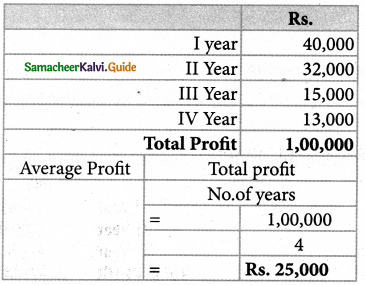Calculation of Goodwill:
Goodwill = Average Profit × two years purchase
= 25,000 × 2
= Rs. 50,000.

Question 2.
Three year’s purchase of the last four years average profits is eed the value of goodwill. The profits and losses for the last four years are: I year Rs. 50,000, II year Rs. 80,000; III year Rs. 30,000 (Loss): IV year Rs. 60,000.
Calculate the amount of goodwill.
Solution:
a) Calculation of average Profit:

 Rs. I year 50,000 II Year 80,000 IV Year 60,000 Profit 3 Years 1,90,000 III Year Loss 30,000 Total Profit 1,60,000

Total profit No. of years 1,60,000Calculation of Goodwill:
Goodwill = Average Profit × three year’s purchase
= 40,000 × 3
= Rs. 1,20,000Question 3.
A finis’:-‘ net profits durL the last three years were Rs. 90,000, Rs. 1,00,000 and Rs. 1,10,000. The capital employed in the firm is Rs. 3,00,000. A normal return on the capital is 10%. Calculate the value of goodwill on the basis of two year’s pruchase of super p it.
Solution:
a) Calculation of average Profit:

 Rs. I year 90,000 II Year 1,00,000 III Year 1,10,000 Total Profit 3,00,000Calculation of Super Profit:
Sceper Profit = Average Profit – Normal Profit
= 1,00,000 – 30,000
= Rs. 70,000

Goodwill at two years purchase of super profit:
Goodwill = Super Profit × No. of years of purchase
= 70,000 × 2
= Rs. 1,40,000

Question 4.
Goodwill is to be valued at three years purchase of five year’s average profits. The profits – for the last five years of the firm were: 2,000-Rs.4,200; 2001-Rs.4,500; 2002-Rs.4,700; 2003-Rs.4,600; and 2004-Rs.5,000.
Solution
G/W = Average Profits x No. of yrs of purchase
Average Profit = $$\frac{4200+4500+4700+4600+5000}{5}$$
Average Profit = $$\frac{23,000}{5}$$
= Rs. 4,600
G/W = 4,600 × 3yrs
= Rs. 13,800Question 5.
Calculate the amount of goodwill on the basis of two year’s purchase of the last four years average profits. The profits for the last four year’s are:

 Rs. I year Loss 10,000 II Year Profit 26,000 III Year Profit 34,000 IV Year Profit 50,000

Solution:
Average Profit = $$\frac{26,000+34,000+50,000-10,000}{4}$$
Average Profit = 25,000
G/W = Average Profits × No. of yrs of purchase
G/W = 25,000 × 2
= Rs. 50,000

Question 6.
A firm earned net profits during the last three years as follows:

 Rs. I year 36,000 II Year 40,000 III Year 44,000

The Capital inverstment of the firm is Rs. 1,20,000. A fair return on the capital having regard to the risk involved in 10% calculate the value of goodwill on the basis of three years purchase of super profits.
Solution:1,20,000 x $$\frac{10}{100}$$ = Rs. 12,000
Super Profit = Average profit – Normal profit
= 40,000 – 12,000
Rs. 28,000
G/w = Super profit × No. of. yrs of purchase
= 28,000 × 3 years.
G/w = Rs. 84,000Question 7.
From the following information, calculate the value of goodwill at three year’s purchase of super profit.
Average Capital employed in the business Rs. 6,00,000
Net trading profits of the firm for the past three years were Rs. .1,07,600, Rs. 90,700 and Rs. 1,12,500.
Rate of interest expected from capital having to the risk involved is 12%.
Fair remuneration to the partners for their service Rs. 12,000 p.a.
Solution:
Average Profit = $$\frac{1,07,600+90,700+1,12,500}{3}$$
Average Profit = $$\frac{3,10,800}{3}$$= Rs. 1,03,600
Profit before adj of renumeration = Rs. 1,03,600
Less Remineration = 12,000
Avg. Profit = 91,600
Normal Profit = $$\frac{\text { Cap. Emp } \times \text { Normal rate }}{100}$$
= $$6,00,000 \times \frac{12}{100}$$
= Rs. 72,000

Super Profit = Average Profit – Normal Profit
= 91,600 – 72,000
= Rs. 19,600

G/w = Super profit x No. of yrs of purchase
= 19,600 × 3yrs
= Rs. 58,800